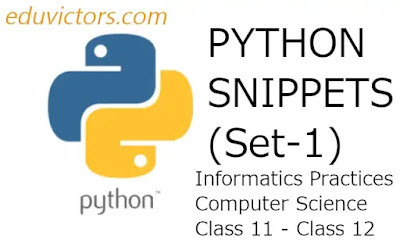# Python Snippets For Class 11 and Class 12

List of Python programs for students studying Informatics Practices and Computer Science.

Program 1: Write a program in python to accept a string and display it in reverse order.

`# write a program in python to accept a string and display it in a reverse order.str1=input("Enter a string: ")print("Reverse string is: ",str1[::-1])`

Output:

Program 2: Write a program in python to extract a list slice out of a given list of numbers from 1 to 20. Display average of elements in the slice that contains every fourth element of the list.

```#Write a program in python to extract a list slice out of a given list of numbers # from 1 to 20. Display average of elements# in the slice that contains every fourth# element of the list.
a = []for i in range(1,21):a.append(i)sum = 0for element in a[::4]:sum+=elementprint(sum/5)```

Output:

Program 3:Write a program in python to accept a string and check whether it is palindrome or not.

```#Write a program in python to accept a string and check
#whether it is palindrome or not.

word=input("Enter a string: ")
reverse_word = ''
for ch in word:
reverse_word = ch + reverse_word
if reverse_word == word:
print(f'{word} is a palindrome.')
else:
print(f'{word} is not a palindrome.')
```

Output:

Program 4:Write a program in python to calculate mean of a list of numbers.

```#Write a program in python to calculate mean of a list of numbers.
l=[1,2,3,4,5]
sum = 0
count = 0
for element in l:
sum+=element
count+=1
print(sum/count)
```

Output: